# MCAT Math Practice Test 3: Data-Based and Statistical Reasoning

### Test Information15 questions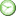minutes

Take more free MCAT math practice tests available from maintests.com.

1. Which of the following outliers would most likely be the easiest to correct?

• A. A typographical error in data transfer
• B. A measurement error in instrument calibration
• C. A heavily skewed distribution
• D. A correctly measured anomalous result

2. In a sample of hospital patients, the mean age is found to be significantly lower than the median. Which of the following best describes this distribution?

• A. Skewed right
• B. Skewed left
• C. Normal distribution
• D. Bimodal distribution

3. What is the median of the following data set?

7, 17, 53, 23, 4, 2, 4

• A. 4
• B. 7
• C. 15.7
• D. 23

4. A hypothesis test was correctly conducted and the experimenter failed to reject the null hypothesis. Which of the following must be true?

I. The p-value was greater than α.

II. A type I error did not occur.

III. The power of the study was too small.

• A. I only
• B. II only
• C. I and II only
• D. I and III only

5. A 95% confidence interval will fall within what distance from the mean?

• A. ±σ
• B. ±2σ
• C. ±3σ
• D. ±4σ

6. Are there any outliers on the following box plot?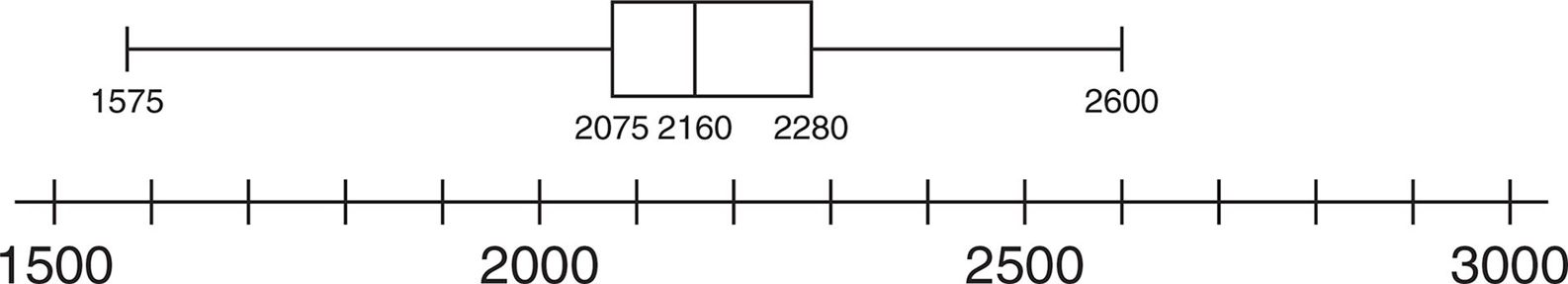• A. Yes; 1575 is an outlier.
• B. Yes; 2600 is an outlier.
• C. Yes; both 1575 and 2600 are outliers.
• D. No; there are no outliers.

7. The following titration curve is an example of: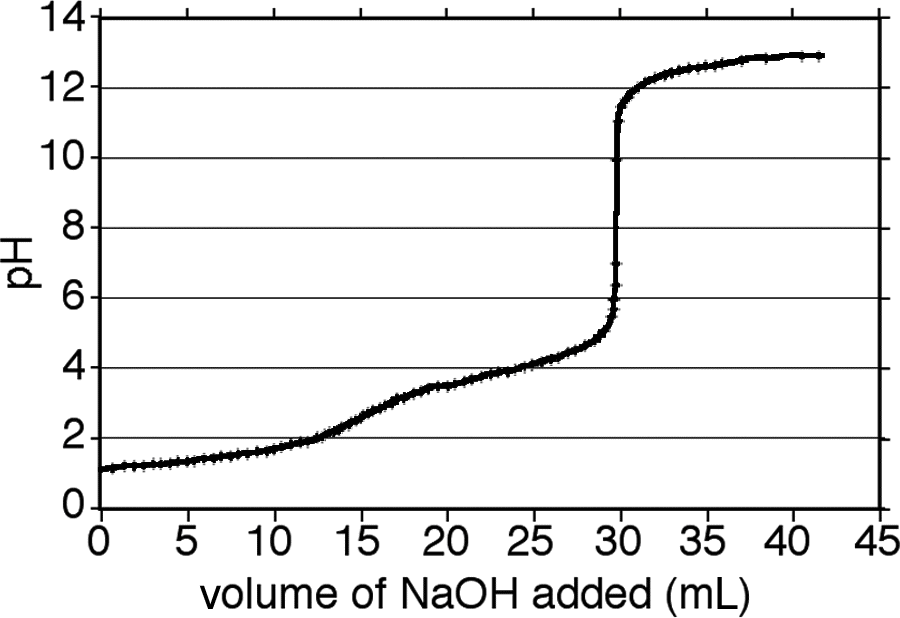• A. a sigmoidal relationship on a log–log graph.
• B. a sigmoidal relationship on a linear graph.
• C. a logarithmic relationship on a semilog graph.
• D. a logarithmic relationship on a log–log graph.

8. Assume that having blonde hair and blue eyes are independent recessive traits. If one parent is a carrier for each gene while the other parent is homozygous recessive for both genes, what is the probability that the first two offspring will both have blonde hair and blue eyes?

• A. 6.25%
• B. 25%
• C. 43.75%
• D. 50%

9. Based on the county-level map below, which of the following statements best represents the data about elderly individuals? (Note: The darker the shade of green, the higher the percentage of elderly persons in the county.)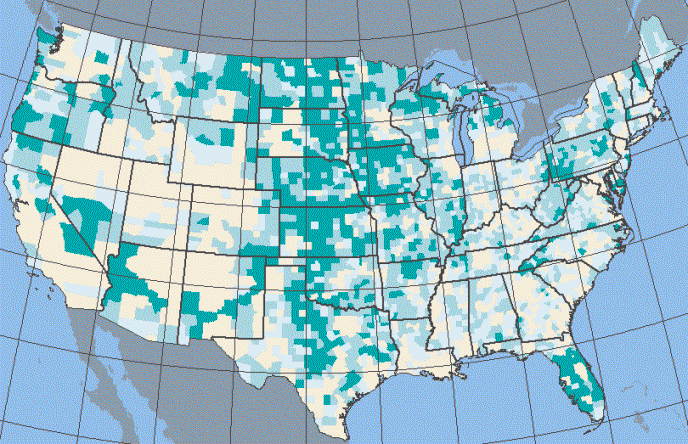• A. Most of the elderly people in the United States live in the center of the country.
• B. Most of the people living in the center of the United States are elderly.
• C. The center of the United States tends to have a larger proportion of elderly people.
• D. There are more elderly people moving to the center of the country than elsewhere.

10. As the confidence level increases, a confidence interval:

• A. becomes wider.
• B. becomes thinner.
• C. shifts to higher values.
• D. shifts to lower values.

11. Which of the following measures of distribution is most useful for determining probabilities?

• A. Range
• B. Average distance from mean
• C. Interquartile range
• D. Standard deviation

12. It is known that crickets increase their rate of chirping in a direct linear relationship with temperature until a maximum chirping rate is reached. Which of the following graphs best represents this relationship?

• A.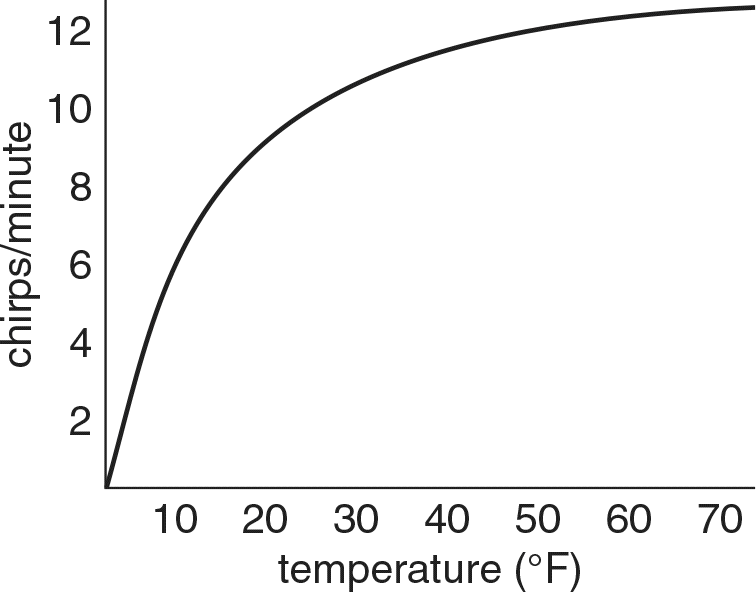• B.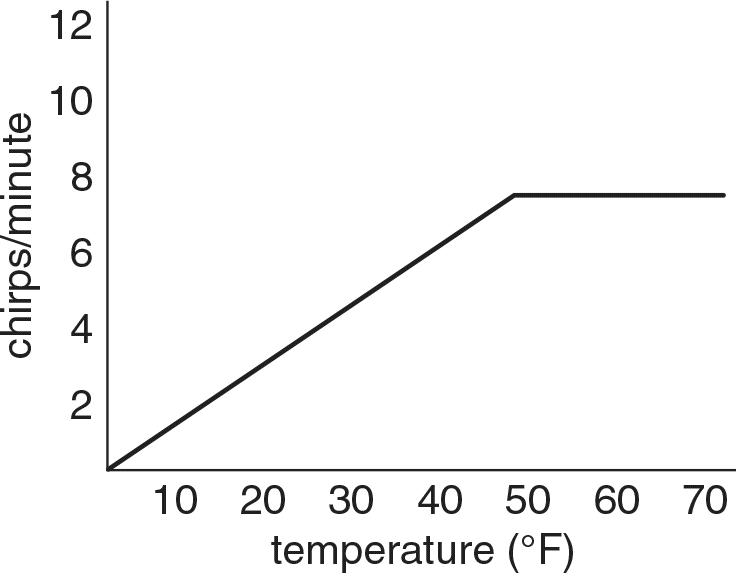• C.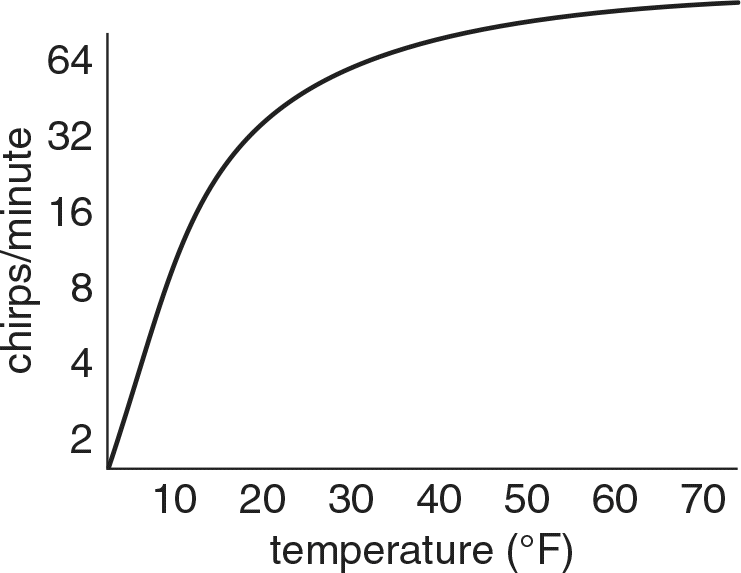• D.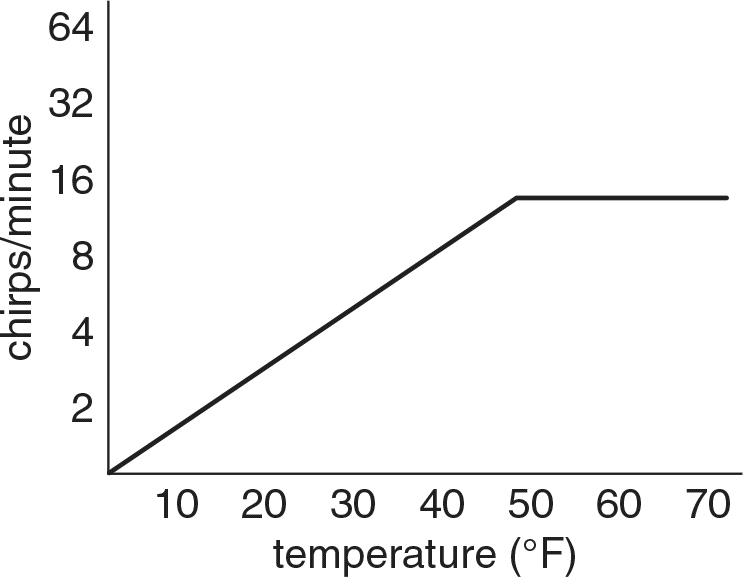13. A new medication for heart failure is being developed and has had a statistically significant effect on contractility in clinical trials. Which of the following would NOT likely cause the drug to be held back from common use?

• A. The value of α used was 0.5.
• B. Similar compounds display toxicity.
• C. The effect size is clinically insignificant.
• D. The study had low power to detect a difference.

14. The following histogram: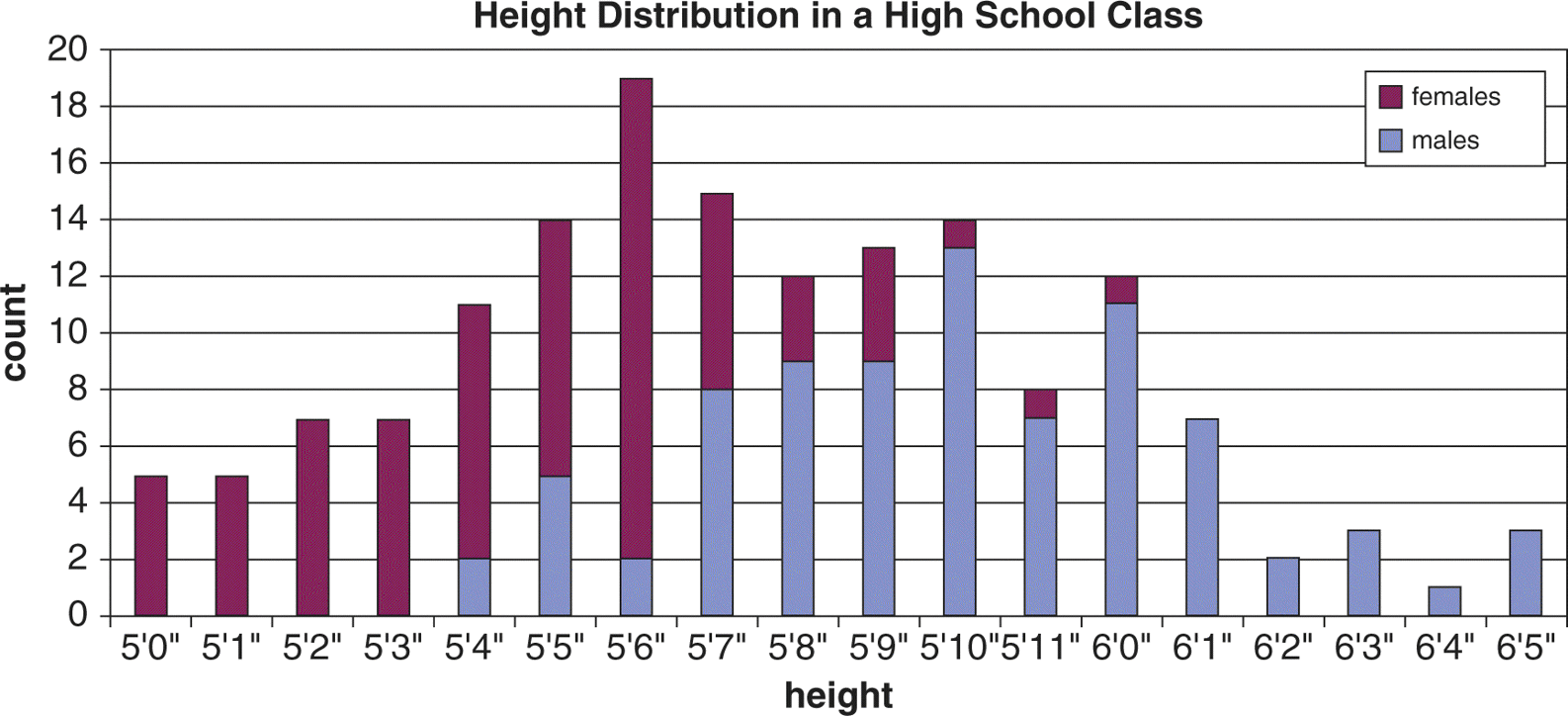I. contains a bimodal distribution.

II. should be analyzed as two separate distributions.

III. contains one mode.

• A. II only
• B. I and II only
• C. I and III only
• D. I, II, and III

15. Which of the following values corresponds to the probability of a type I error?

• A. α
• B. β
• C. Power
• D. Confidence

﻿